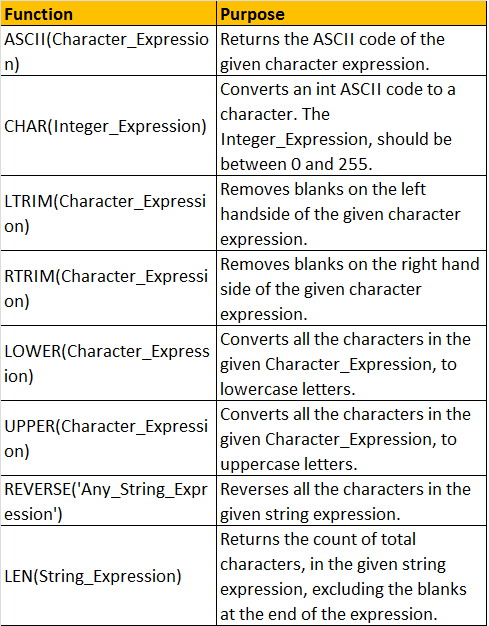HI WELCOME TO SIRING

# Built in string functions in sql server 2008 - Part 22

Functions in SQL server can be broadly divided into 2 categoris
1. Built-in functions
2. User Defined functions

There are several built-in functions. In this video session, we will look at the most common string functions available.

ASCII(Character_Expression) - Returns the ASCII code of the given character expression.
To find the ACII Code of capital letter 'A'

Example:
Select ASCII('A')

Output: 65

CHAR(Integer_Expression) - Converts an int ASCII code to a character. The Integer_Expression, should be between 0 and 255.
The following SQL, prints all the characters for the ASCII values from o thru 255

Declare @Number int

Set @Number = 1
While(@Number <= 255)
Begin
Print CHAR(@Number)
Set @Number = @Number + 1
End

Note: The while loop will become an infinite loop, if you forget to include the following line.
Set @Number = @Number + 1

Printing uppercase alphabets using CHAR() function:
Declare @Number int
Set @Number = 65
While(@Number <= 90)
Begin
Print CHAR(@Number)
Set @Number = @Number + 1
End

Printing lowercase alphabets using CHAR() function:
Declare @Number int
Set @Number = 97
While(@Number <= 122)
Begin
Print CHAR(@Number)
Set @Number = @Number + 1
End

Another way of printing lower case alphabets using CHAR() and LOWER() functions.
Declare @Number int
Set @Number = 65
While(@Number <= 90)
Begin
Print LOWER(CHAR(@Number))
Set @Number = @Number + 1
End

LTRIM(Character_Expression) - Removes blanks on the left handside of the given character expression.

Example: Removing the 3 white spaces on the left hand side of the '   Hello' string using LTRIM() function.
Select LTRIM('   Hello')
Output: Hello

RTRIM(Character_Expression) - Removes blanks on the right hand side of the given character expression.

Example
: Removing the 3 white spaces on the left hand side of the 'Hello   ' string using RTRIM() function.

Select RTRIM('Hello   ')
Output: Hello

Example: To remove white spaces on either sides of the given character expression, use LTRIM() and RTRIM() as shown below.
Select LTRIM(RTRIM('   Hello   '))
Output: Hello

LOWER(Character_Expression) - Converts all the characters in the given Character_Expression, to lowercase letters.

ExampleSelect LOWER('CONVERT This String Into Lower Case')

Output: convert this string into lower case

UPPER(Character_Expression) - Converts all the characters in the given Character_Expression, to uppercase letters.
ExampleSelect UPPER('CONVERT This String Into upper Case')
Output: CONVERT THIS STRING INTO UPPER CASE

REVERSE('Any_String_Expression') - Reverses all the characters in the given string expression.
ExampleSelect REVERSE('ABCDEFGHIJKLMNOPQRSTUVWXYZ')
Output: ZYXWVUTSRQPONMLKJIHGFEDCBA

LEN(String_Expression) - Returns the count of total characters, in the given string expression, excluding the blanks at the end of the expression.

ExampleSelect LEN('SQL Functions   ')

Output: 13In the next video session, we will discuss about the rest of the commonly used built-in string functions.